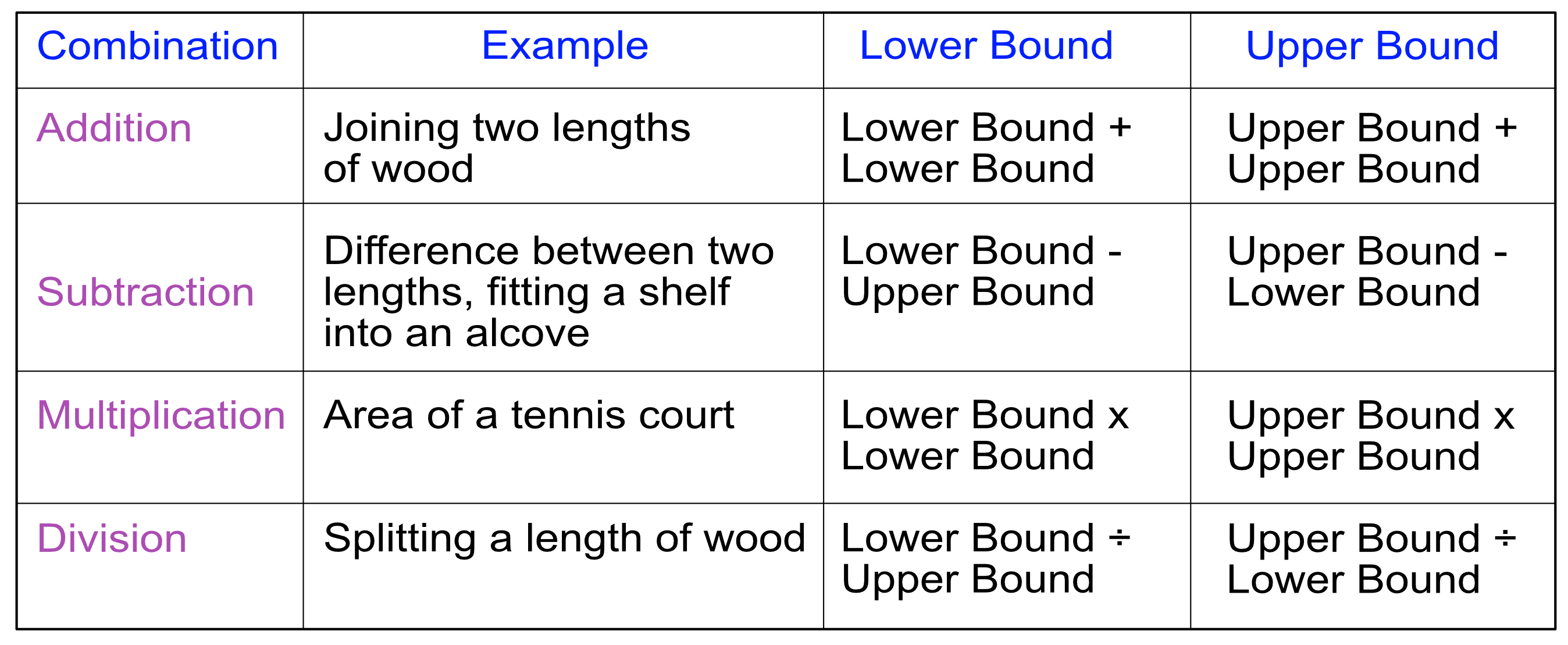Upper and Lower Bounds

# Upper and Lower Bounds

GCSE(H)

When combining upper and lower bounds, it is necessary to work out how the measurements relate to one another.## Examples

1. A shelf is being fitted into an alcove. The shelf is 2.3m long, with an error interval of 5cm; and the alcove is 2.4m long, with an error interval of 5cm. What is the biggest gap that there might be when fitting the shelf to the alcove?

The gap is given by the smallest shelf size and the largest alcove size.

Shelf lower bound: 2.3 - 0.05 ÷ 2 = 2.275

Alcove Upper Bound = 2.4 + 0.05 ÷ 2 = 2.425

Difference = 2.425 - 2.275 = 0.15m

2. Crates are being loaded onto a pallet for lifting by a crane. The maximum weight the crane can lift is 1000kg. Each crate weighs 85kg, to the nearest kg. What is the maximum number of crates to go on the pallet?